# Electric Potential Electric Potential The electric potential difference

• Slides: 9Electric Potential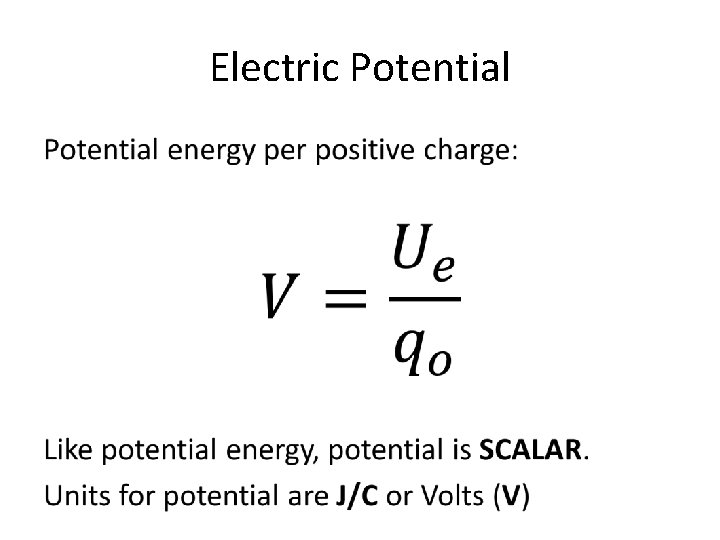Electric Potential •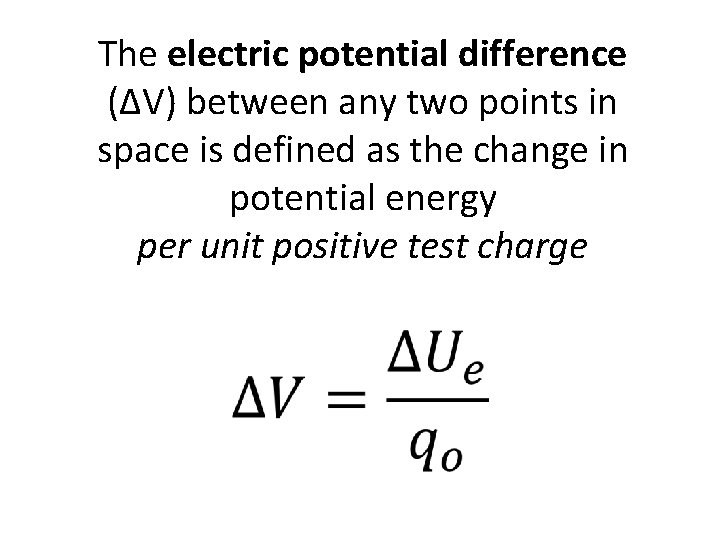The electric potential difference (ΔV) between any two points in space is defined as the change in potential energy per unit positive test charge •Formulas •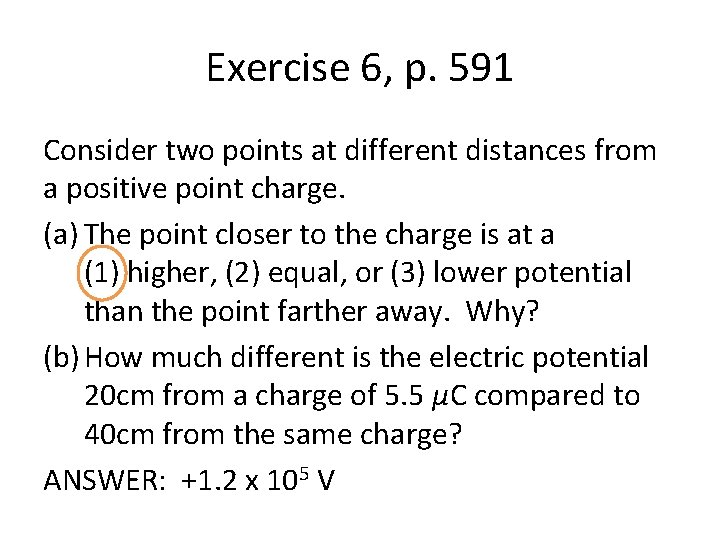Exercise 6, p. 591 Consider two points at different distances from a positive point charge. (a) The point closer to the charge is at a (1) higher, (2) equal, or (3) lower potential than the point farther away. Why? (b) How much different is the electric potential 20 cm from a charge of 5. 5 µC compared to 40 cm from the same charge? ANSWER: +1. 2 x 105 V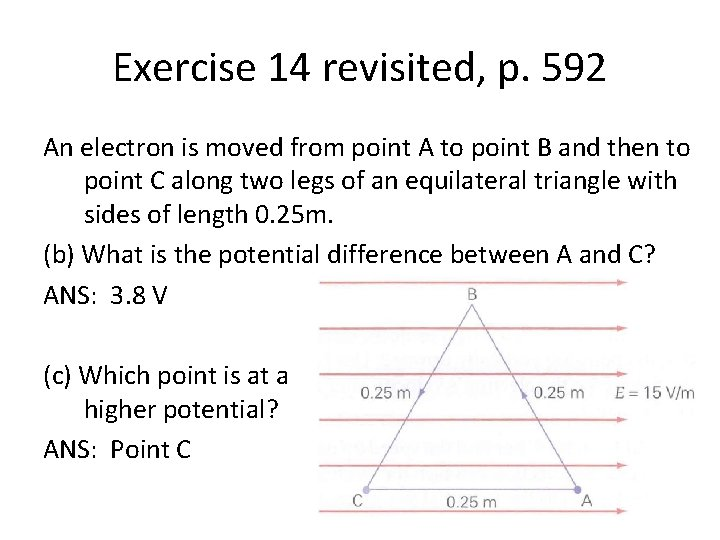Exercise 14 revisited, p. 592 An electron is moved from point A to point B and then to point C along two legs of an equilateral triangle with sides of length 0. 25 m. (b) What is the potential difference between A and C? ANS: 3. 8 V (c) Which point is at a higher potential? ANS: Point CThree charges on a line Find the potential at location 1. ANSWER: -6 x 105 V 2 -4. 0μC 0. 2 m 1 0. 15 m 3 -7. 0 μC Find the potential energy of a +3. 0 µC placed at location 1. ANSWER: -1. 8 J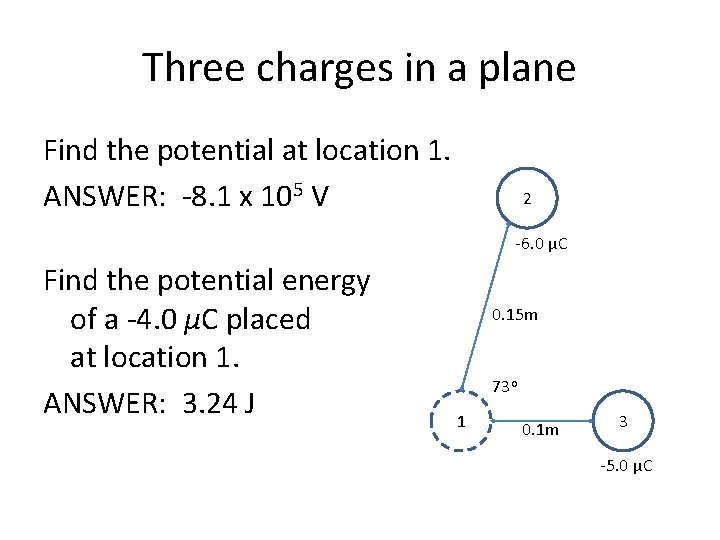Three charges in a plane Find the potential at location 1. ANSWER: -8. 1 x 105 V 2 -6. 0 μC Find the potential energy of a -4. 0 µC placed at location 1. ANSWER: 3. 24 J 0. 15 m 73 o 1 0. 1 m 3 -5. 0 μC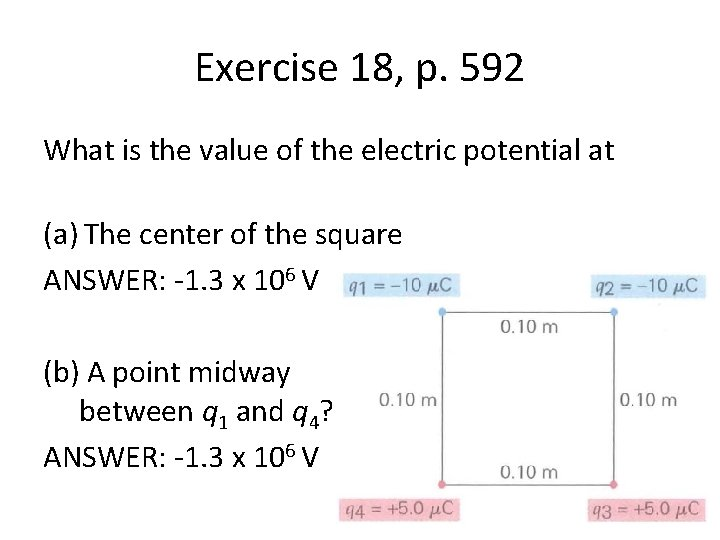Exercise 18, p. 592 What is the value of the electric potential at (a) The center of the square ANSWER: -1. 3 x 106 V (b) A point midway between q 1 and q 4? ANSWER: -1. 3 x 106 V